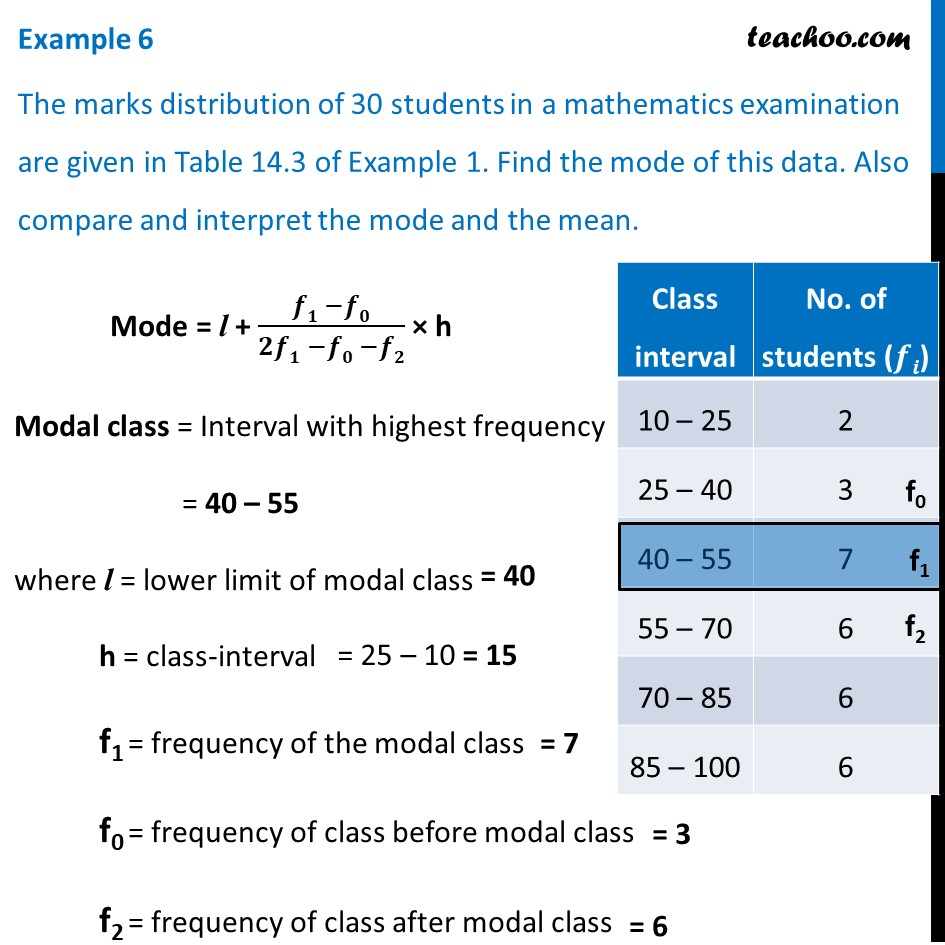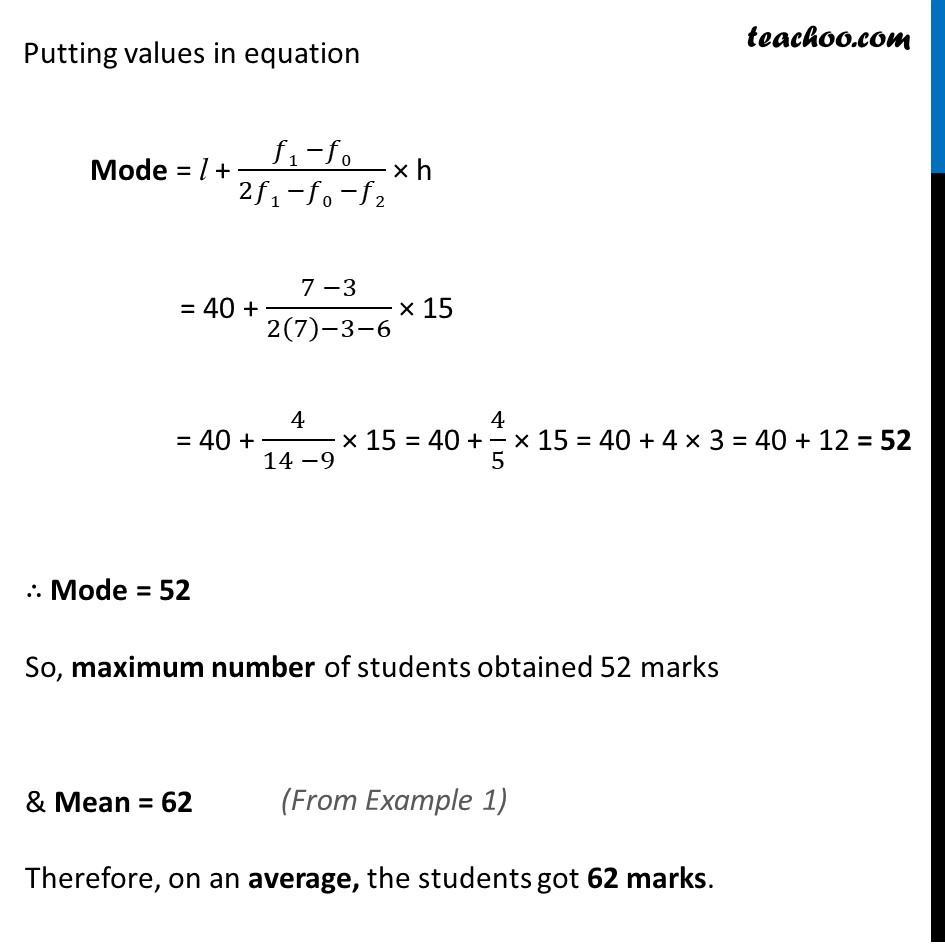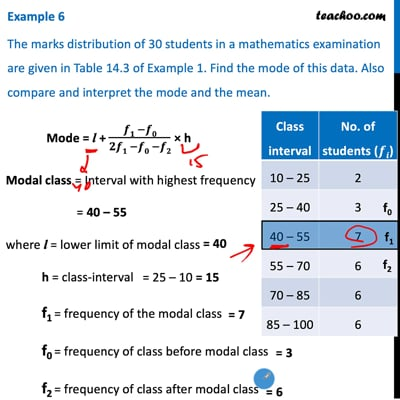Examples

Chapter 14 Class 10 Statistics
Serial order wiseThis video is only available for Teachoo black users

Solve all your doubts with Teachoo Black (new monthly pack available now!)

### Transcript

Example 6 The marks distribution of 30 students in a mathematics examination are given in Table 14.3 of Example 1. Find the mode of this data. Also compare and interpret the mode and the mean. Mode = l + (𝒇𝟏 −𝒇𝟎)/(𝟐𝒇𝟏 −𝒇𝟎 −𝒇𝟐) × h Modal class = Interval with highest frequency = 40 – 55 where l = lower limit of modal class h = class-interval f1 = frequency of the modal class f0 = frequency of class before modal class f2 = frequency of class after modal class Putting values in equation Mode = l + (𝑓1 −𝑓0)/(2𝑓1 −𝑓0 −𝑓2) × h = 40 + (7 −3)/(2(7)−3−6) × 15 = 40 + 4/(14 −9) × 15 = 40 + 4/5 × 15 = 40 + 4 × 3 = 40 + 12 = 52 ∴ Mode = 52 So, maximum number of students obtained 52 marks & Mean = 62 Therefore, on an average, the students got 62 marks.